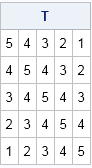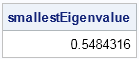Occasionally a SAS statistical programmer will ask me, "How can I construct a large correlation matrix?" Often they are simulating data with SAS or developing a matrix algorithm that involves a correlation matrix. Typically they want a correlation matrix that is too large to input by hand, such as a correlation matrix for 100 variables. The particular correlations are not usually important; they just want any valid correlation matrix.

Except for diagonal and diagonally dominant matrices, it is not easy to write down a valid correlation matrix. I've previously written about the fact that not every symmetric matrix with a unit diagonal is a correlation matrix. Correlation matrices are symmetric and positive definite (PD), which means that all the eigenvalues of the matrix are positive. (Technically, a correlation matrix can have a zero eigenvalues, but that is a degenerate case that I prefer to avoid.)

So here is a tip: you can generate a large correlation matrix by using a special Toeplitz matrix.

Recall that a Toeplitz matrix has a banded structure. The diagonals that are parallel to the main diagonal are constant. The SAS/IML language has a built-in TOEPLITZ function that returns a Toeplitz matrix, as shown:

```proc iml; T = toeplitz(5:1); /* 5x5 Toeplitz matrix */ print T;```A Toeplitz matrix is obviously symmetric, and the following computation shows that the smallest eigenvalue is positive, which means that the matrix is positive definite:

```smallestEigenvalue = min( eigval(T) ); /* compute smallest eigenvalue */ print smallestEigenvalue;```### Generating positive definite Toeplitz matrices

In the previous example, the matrix was generated by the vector {5,4,3,2,1}. Because it is symmetric and PD, it is a valid covariance matrix. Equivalently, the scaled Toeplitz matrix that is generated by the vector {1,0.8,0.6,0.4,0.2} is a correlation matrix that is also PD. This example is a specific case of a very cool mathematical fact:

A Toeplitz matrix generated from any linearly decreasing sequence of nonnegative values is positive definite.

In other words, for every positive number R and increment h, the k-element vector {R, R-h, R-2h, ..., R-(k-1)h} generates a valid covariance matrix provided that R-(k-1)h > 0, which is equivalent to hR/(k-1). A convenient choice is h = R / k. This is a useful fact because it enables you to construct arbitrarily large Toeplitz matrices from a decreasing sequence. For example, the following statements uses R=1 and h=0.01 to construct a 100 x 100 correlation matrix. You can use the matrix to simulate data from a multivariate normal correlated distribution with 100 variables.

```p = 100; h = 1/p; /* stepsize for decreasing sequence */ v = do(1, h, -h); /* {1, 0.99, 0.98, ..., 0.01} */ Sigma = toeplitz(v); /* PD correlation matrix */   mu = j(1, ncol(v), 0); /* population mean vector: (0,0,0,...0) */ X = randnormal(500, mu, Sigma); /* generate 500 obs from MVN(mu, Sigma) */```

A proof of the fact that the Toeplitz matrix is PD is in a paper by Bogoya, Böttcher, and Grudsky (J. Spectral Theory, 2012), who prove the result on p. 273.

### A stronger result: Allowing negative correlations

In fact, Bogoya, Böttcher, and Grudsky prove a stronger result. The previous example assumes that all 100 variables are positively correlated with each other. The paper also proves that you can use a decreasing sequence of length n that contains negative values, as long as the sum of the values is positive. Thus to generate 100 variables with almost as many negative correlations as positive, you can choose h = 2/p, as follows:

```h = 2/p; /* stepsize for decreasing sequence */ v = do(1, -1+h, -h); /* {1, 0.98, 0.96, ..., -0.96, -0.98} */ Sigma = toeplitz(v); /* PD correlation matrix */```

### Large covariance matrices

A Toeplitz matrix creates a covariance matrix that has a constant diagonal, which corresponds to having the same variance for all variables. However, you can use the CORR2COV function in SAS/IML to convert a correlation matrix to a covariance matrix. Algebraically, this transformation is accomplished by pre- and post-multiplying by a diagonal matrix, which will preserve positive definiteness. For example, the following statement converts Sigma into a covariance matrix for which each variable has a different variance:

```sd = 1:p; Cov = corr2cov(Sigma, sd); /* make std(x_i) = i */```

The next time you need to generate a large symmetric positive-definite matrix, remember the Toeplitz matrix.

ShareDistinguished Researcher in Computational Statistics

Rick Wicklin, PhD, is a distinguished researcher in computational statistics at SAS and is a principal developer of SAS/IML software. His areas of expertise include computational statistics, simulation, statistical graphics, and modern methods in statistical data analysis. Rick is author of the books Statistical Programming with SAS/IML Software and Simulating Data with SAS.

1.A naive question, but wouldn't it be possible to generate the positive semidefinite matrix as a positive weighted sum of rank-1 matrices? That way the sparsity can also be controlled.

•Yes, it is possible, since each x`*x is PSD, but I don't see it as very helpful. A primary purpose for wanting to create a large PD matrix is so that you can can simulate vectors from MVN (or other multivariate distribution). If you form a PSD matrix by using n linearly independent vectors, how do you obtain those vectors? If you choose independent vectors, the correlation matrix will always be close to the identity matrix, which isn't very interesting. In general, for arbitrary vectors, you won't be able to predict what the correlation matrix will look like. The article's method enables you to specify the values of the correlation matrix, which is a powerful property to control.

2.i have my correlation matrix like this [0.5, 10^-5 i ; 10^-5 i, 0.5], how can i create Toeplitz matrix for Circulant embedding method.

•I am not an expert on complex-valued random variables. I suggest you ask your question on CrossValidated.